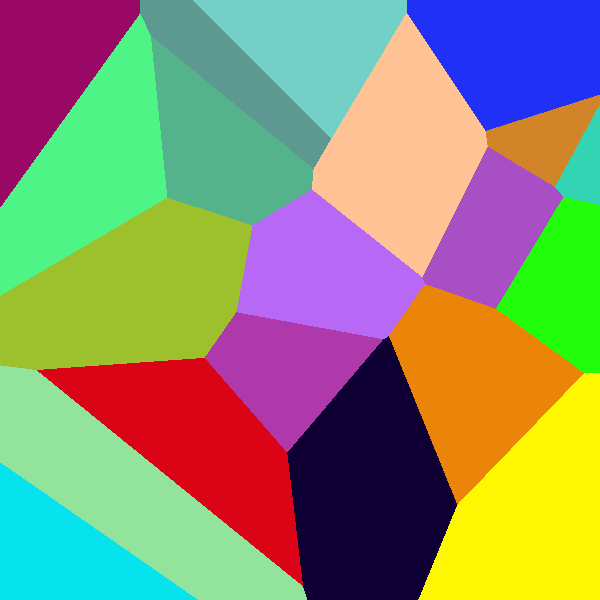﻿ voronoi – Algosim documentation
Algosim documentation: voronoi

# voronoi

Creates a Voronoi diagram.

## Syntax

• `voronoi(w, h, S, C)`

• `w` and `h` are positive integers

• `S` is a list of points

• `C` is a list of colours

## Description

If `w` and `h` are positive integers, `S` a list of integer points, and `C` a list of colours of the same length as `S`, then `voronoi(w, h, S, C)` returns a Voronoi diagram of width `w` and height `h` with sites `S` and associated site colours `C`.

## Examples

```w ≔ 600; n ≔ 20;
voronoi(w, w, compute('(RandomInt(w), RandomInt(w)), i, 1, n), compute(RandomColor(), i, 1, n))
```